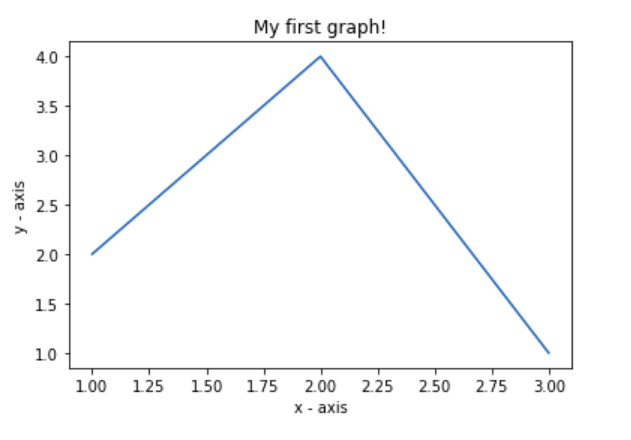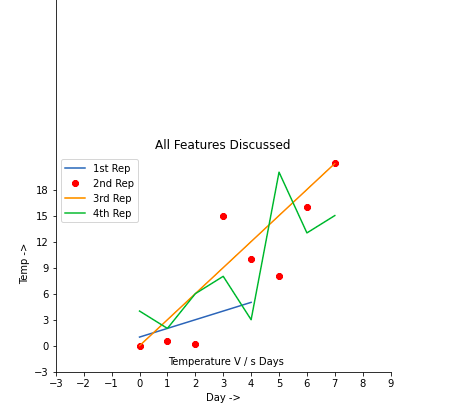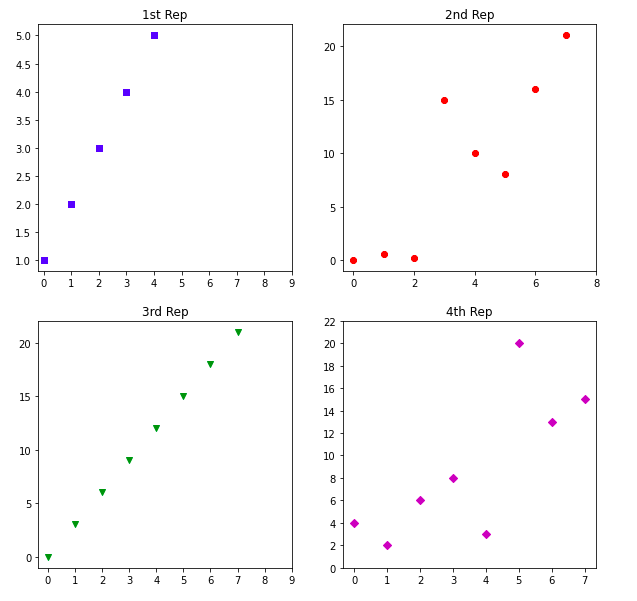Related Articles

# Simple Plot in Python using Matplotlib

• Difficulty Level : Medium
• Last Updated : 30 Apr, 2020

Matplotlib is a Python library that helps in visualizing and analyzing the data and helps in better understanding of the data with the help of graphical, pictorial visualizations that can be simulated using the matplotlib library. Matplotlib is a comprehensive library for static, animated and interactive visualizations.

### Installation of matplotlib library

Step 1: Open command manager (just type “cmd” in your windows start search bar)
Step 2: Type the below command in the terminal.

`cd Desktop`

Step 3: Then type the following command.

`pip install matplotlib`

## Creating a Simple Plot

 `# importing the required module ``import` `matplotlib.pyplot as plt ``   ` `# x axis values ``x ``=` `[``1``,``2``,``3``] ``# corresponding y axis values ``y ``=` `[``2``,``4``,``1``] ``   ` `# plotting the points  ``plt.plot(x, y) ``   ` `# naming the x axis ``plt.xlabel(``'x - axis'``) ``# naming the y axis ``plt.ylabel(``'y - axis'``) ``   ` `# giving a title to my graph ``plt.title(``'My first graph!'``) ``   ` `# function to show the plot ``plt.show() `

Output:The code seems self-explanatory. Following steps were followed:

• Define the x-axis and corresponding y-axis values as lists.
• Plot them on canvas using .plot() function.
• Give a name to x-axis and y-axis using .xlabel() and .ylabel() functions.
• Give a title to your plot using .title() function.
• Finally, to view your plot, we use .show() function.

Let’s have a look at some of the basic functions that are often used in matplotlib.

MethodDescription
plot()it creates the plot at the background of computer, it doesn’t displays it. We can also add a label as it’s argument that by what name we will call this plot – utilized in legend()
show()it displays the created plots
xlabel()it labels the x-axis
ylabel()it labels the y-axis
title()it gives the title to the graph
gca()it helps to get access over the all the four axes of the graph
gca().spines[‘right/left/top/bottom’].set_visible(True/False)it access the individual spines or the individual boundaries and helps to change theoir visibility
xticks()it decides how the markings are to be made on the x-axis
yticks()it decides how the markings are to be made on the y-axis
gca().legend()pass a list as it’s arguments of all the plots made, if labels are not explicitly specified then add the values in the list in the same order as the plots are made
annotate()it is use to write comments on the graph at the specified position
figure(figsize = (x, y))whenever we want the result to be displayed in a separate window we use this command, and figsize argument decides what will be the initial size of the window that will be displayed after the run
subplot(r, c, i)it is used to create multiple plots in the same figure with r signifies the no of rows in the figure, c signifies no of columns in a figure and i specifies the positioning of the particular plot
set_xticksit is used to set the range and the step size of the markings on x – axis in a subplot
set_yticksit is used to set the range and the step size of the markings on y – axis in a subplot

Note: Try removing the features added one by one and understand how does the output result changes

Example 1:

 `import` `matplotlib.pyplot as plt`` ` ` ` `a ``=` `[``1``, ``2``, ``3``, ``4``, ``5``]``b ``=` `[``0``, ``0.6``, ``0.2``, ``15``, ``10``, ``8``, ``16``, ``21``]``plt.plot(a)`` ` `# o is for circles and r is ``# for red``plt.plot(b, ``"or"``)`` ` `plt.plot(``list``(``range``(``0``, ``22``, ``3``)))`` ` `# naming the x-axis``plt.xlabel(``'Day ->'``)`` ` `# naming the y-axis``plt.ylabel(``'Temp ->'``)`` ` `c ``=` `[``4``, ``2``, ``6``, ``8``, ``3``, ``20``, ``13``, ``15``]``plt.plot(c, label ``=` `'4th Rep'``)`` ` `# get current axes command``ax ``=` `plt.gca()`` ` `# get command over the individual``# boundary line of the graph body``ax.spines[``'right'``].set_visible(``False``)``ax.spines[``'top'``].set_visible(``False``)`` ` `# set the range or the bounds of ``# the left boundary line to fixed range``ax.spines[``'left'``].set_bounds(``-``3``, ``40``)`` ` `# set the interval by  which ``# the x-axis set the marks``plt.xticks(``list``(``range``(``-``3``, ``10``)))`` ` `# set the intervals by which y-axis``# set the marks``plt.yticks(``list``(``range``(``-``3``, ``20``, ``3``)))`` ` `# legend denotes that what color ``# signifies what``ax.legend([``'1st Rep'``, ``'2nd Rep'``, ``'3rd Rep'``, ``'4th Rep'``])`` ` `# annotate command helps to write``# ON THE GRAPH any text xy denotes ``# the position on the graph``plt.annotate(``'Temperature V / s Days'``, xy ``=` `(``1.01``, ``-``2.15``))`` ` `# gives a title to the Graph``plt.title(``'All Features Discussed'``)`` ` `plt.show()`

Output:Example 2:

 `import` `matplotlib.pyplot as plt`` ` ` ` `a ``=` `[``1``, ``2``, ``3``, ``4``, ``5``]``b ``=` `[``0``, ``0.6``, ``0.2``, ``15``, ``10``, ``8``, ``16``, ``21``]``c ``=` `[``4``, ``2``, ``6``, ``8``, ``3``, ``20``, ``13``, ``15``]`` ` `# use fig whenever u want the ``# output in a new window also ``# specify the window size you``# want ans to be displayed``fig ``=` `plt.figure(figsize ``=``(``10``, ``10``))`` ` `# creating multiple plots in a ``# single plot``sub1 ``=` `plt.subplot(``2``, ``2``, ``1``)``sub2 ``=` `plt.subplot(``2``, ``2``, ``2``)``sub3 ``=` `plt.subplot(``2``, ``2``, ``3``)``sub4 ``=` `plt.subplot(``2``, ``2``, ``4``)`` ` `sub1.plot(a, ``'sb'``)`` ` `# sets how the display subplot ``# x axis values advances by 1``# within the specified range``sub1.set_xticks(``list``(``range``(``0``, ``10``, ``1``)))``sub1.set_title(``'1st Rep'``)`` ` `sub2.plot(b, ``'or'``)`` ` `# sets how the display subplot x axis``# values advances by 2 within the``# specified range``sub2.set_xticks(``list``(``range``(``0``, ``10``, ``2``)))``sub2.set_title(``'2nd Rep'``)`` ` `# can directly pass a list in the plot``# function instead adding the reference``sub3.plot(``list``(``range``(``0``, ``22``, ``3``)), ``'vg'``)``sub3.set_xticks(``list``(``range``(``0``, ``10``, ``1``)))``sub3.set_title(``'3rd Rep'``)`` ` `sub4.plot(c, ``'Dm'``)`` ` `# similarly we can set the ticks for ``# the y-axis range(start(inclusive),``# end(exclusive), step)``sub4.set_yticks(``list``(``range``(``0``, ``24``, ``2``)))``sub4.set_title(``'4th Rep'``)`` ` `# without writing plt.show() no plot``# will be visible``plt.show()`

Output:Attention geek! Strengthen your foundations with the Python Programming Foundation Course and learn the basics.

To begin with, your interview preparations Enhance your Data Structures concepts with the Python DS Course. And to begin with your Machine Learning Journey, join the Machine Learning – Basic Level Course

My Personal Notes arrow_drop_up